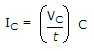# Electronics - Basic Op-Amp Circuits - Discussion

### Discussion :: Basic Op-Amp Circuits - General Questions (Q.No.2)

2.

The formulashows that for a given capacitor, if the voltage changes at a constant rate with respect to time, the current will

 [A]. increase [B]. decrease [C]. be constant [D]. decrease logarithmically

Explanation:

No answer description available for this question.

 Vimal said: (Apr 7, 2011) Integral (Icdt/C) = Vc SO dVc/dt=Ic/t...Constant

 Pols said: (May 24, 2011) Capacitor is open in dc.

 Yugandhar said: (Aug 16, 2011) dv/dt=k then v=kt; Substitute.. i=ktC/t; i=kC; i=constant;

 Shiva Prakash said: (Aug 25, 2011) n^2=a^2+r^2

 Vj Tayal said: (May 12, 2012) Voltage changes at const rate means with time v/t will be const and hence I is const.

 Rama Charan said: (May 24, 2012) Since voltage changes with time, so overall ratio (V/t) will be constant.

 Mervin said: (Feb 11, 2013) In case of capacitor the there will be no change in current for the sudden change in voltage.

 Ayush Kumar Srivastav said: (Aug 27, 2013) Since voltage is change with respect to time then we say that the over all ratio is (v/t) will be constant.

 Priyanka said: (Dec 12, 2014) Since voltage change with time, so overall ratio (v/t) will be constant. i.e current will be constant.

 Anomie said: (May 24, 2019) Voltage is constant C is constant Ic is constant with time. But when Ic is C(dv/dt), Then Ic is going to change with time to 5T.

 Edozi said: (Sep 12, 2019) Yes, since the situation is 'voltage changes at constant rate' the current will be constant also because they are proportional with each other.